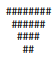# I need help understanding how this loop woks

i want to print the hash symbol in a descending order. that is from 8 to 6 then 4 and 2. but am unable to get the arrangement of the nested loops to work. i have moved the loops around for a lot of time but i cant get the right order https://codepen.io/Aqua7/pen/NWaNZmg?editors=1112

What is your expected output? If the `console.log(row)` is uncommented, it’s printing in console multiple hash characters in the descending order.

What are you trying to do with this code? If all you want is:

``````1111##
111##
11##
1##
``````

Then what you are doing is unnecessarily complicated. This should be solved with a two loops, one nested in the other. It can also be solved with a single loop. You could probably also be solved in a single line, but that might get a little arcane.

1 Like

the expected output is supposed to be thisthe loop below print the string ‘1’ to represent whitespace because I could not get the syntax.

``````for (let i = 4; i >= 1; i--) {
let val = "1";
// i subtracts from nested loop condition when i iterate
for (let j = 1; j < 5 - i; j++) {
val += "1";
}
console.log(val);
}
``````

the code above prints this

``````1
11
111
1111
``````

and then the other loop prints the hash symbol

``````for (let k = 1; k <= 7; k++) {
var row = "";
// k subtracts from nested loop condition everytime k iterate
for (let l = 1; l <= 9 - k; l++) {
row += "#";
}
if (k % 2 === 0) continue;
console.log(row)
}
``````

the code above prints this

``````########
######
####
##
``````

and then I thought of nesting all the loops, thinking it will give me the desired result but it did not. what am i doing wrong, i will still change the ‘1’ output to a whitespace

if you look at the image, you can think of it as a rectangle, 8 columns, 4 rows. You can solve it by using 2 loops, one for creating rows and one for columns(or even one loop which creates rows of 8 symbols each). Where you want fill, you use the hash symbol, where you want blank space, you simply leave space out. With each rows, from top to bottom, you add one additional blank space from left and right(start and end of a row). You can make a counter, which indicates that. Basically the loop iterator can do that, as it starts as 0(no spaces available), continues with 1(1 space of every side), then 2(2 spaces) and so on. You just need to set up the right conditions to whether write “#” or " ".

2 Likes

This topic was automatically closed 182 days after the last reply. New replies are no longer allowed.